# Python 数据挖掘与机器学习实战（五）：基于线性回归的股票预测

• 2020-02-01
• 本文字数：2857 字

阅读完需：约 9 分钟## 3.5　基于线性回归的股票预测

### 3.5.1　数据获取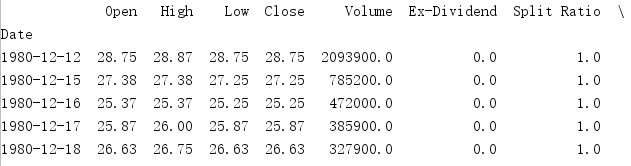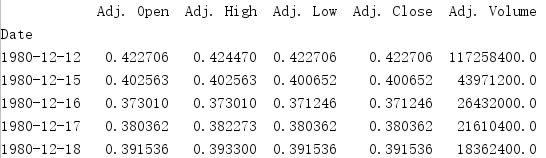### 3.5.2　数据预处理

（股票最高价与最低价变化百分比）：

（股票收盘价与开盘价的变化百分比）：

X = preprocessing.scale(X)

from sklearn.linear_model import LinearRegression

clf = LinearRegression(n_jobs=-1)

clf.fit(X_train, y_train)

forecast_set = clf.predict(X_lately)

accuracy = clf.score(X_test, y_test)

### 3.5.3　编码实现

import quandlfrom sklearn import preprocessing#df = quandl.get('WIKI/GOOGL'),先注释这一行,预测Google股票再用df = quandl.get('WIKI/AAPL')import mathimport numpy as np# 定义预测列变量,它存放研究对象的标签名forecast_col = 'Adj. Close'# 定义预测天数,这里设置为所有数据量长度的1%forecast_out = int(math.ceil(0.01*len(df)))# 只用到df中的下面几个字段df = df[['Adj. Open', 'Adj. High', 'Adj. Low', 'Adj. Close', 'Adj. Volume']]# 构造两个新的列# HL_PCT为股票最高价与最低价的变化百分比df['HL_PCT']=(df['Adj. High'] - df['Adj. Close'])/ df['Adj. Close'] * 100.0# PCT_change为股票收盘价与开盘价的变化百分比df['PCT_change']=(df['Adj.Close']- df['Adj.Open'])/df['Adj. Open'] * 100.0# 下面为真正用到的特征字段df = df[['Adj. Close', 'HL_PCT', 'PCT_change', 'Adj. Volume']]#因为scikit-learn并不会处理空数据,需要把为空的数据都设置为一个比较难出现的值#这里    取-99999,df.fillna(-99999, inplace=True)# 用label代表该字段,是预测结果# 通过让Adj. Close列的数据往前移动1%行来表示df['label'] = df[forecast_col].shift(-forecast_out)# 最后生成真正在模型中使用的数据X?y,以及预测时用到的数据数据X_latelyX = np.array(df.drop(['label'], 1))X = preprocessing.scale(X)# 上面生成label列时留下的最后1%行的数据,这些行并没有label数据,因此可以拿它们    作为预测时用到的输入数据X_lately = X[-forecast_out:]X = X[:-forecast_out]# 抛弃label列中为空的那些行df.dropna(inplace=True)y = np.array(df['label'])# scikit-learn从0.2版本开始废弃cross_validation,改用model_selectionfrom sklearn import  model_selection, svmfrom sklearn.linear_model import LinearRegression# 开始前,先把X和y数据分成两部分,一部分用来训练,一部分用来测试X_train, X_test, y_train, y_test = model_selection.train_test_split(X, y,     test_size=0.2)# 生成scikit-learn的线性回归对象clf = LinearRegression(n_jobs=-1)# 开始训练clf.fit(X_train, y_train)# 用测试数据评估准确性accuracy = clf.score(X_test, y_test)# 进行预测forecast_set = clf.predict(X_lately)print(forecast_set, accuracy)import matplotlib.pyplot as pltfrom matplotlib import styleimport datetime# 修改matplotlib样式style.use('ggplot')one_day = 86400# 在df中新建Forecast列,用于存放预测结果的数据df['Forecast'] = np.nan# 取df最后一行的时间索引last_date = df.iloc[-1].namelast_unix = last_date.timestamp()next_unix = last_unix + one_day# 遍历预测结果,用它向df中追加行# 这些行除了Forecast字段,其他都设为np.nanfor i in forecast_set:    next_date = datetime.datetime.fromtimestamp(next_unix)    next_unix += one_day    # [np.nan for_in range(len(df.columns)-1)]生成不包含Forecast字段的列表    # 而[i]是只包含Forecast值的列表    # 上述两个列表拼接在一起就组成了新行,按日期追加到df的下面    df.loc[next_date] = [np.nan for _ in range(len(df.columns)- 1)] + [i]# 开始绘图df['Adj. Close'].plot()df['Forecast'].plot()plt.legend(loc=4)plt.xlabel('Date')plt.ylabel('Price')plt.show()

### 3.5.4　结果分析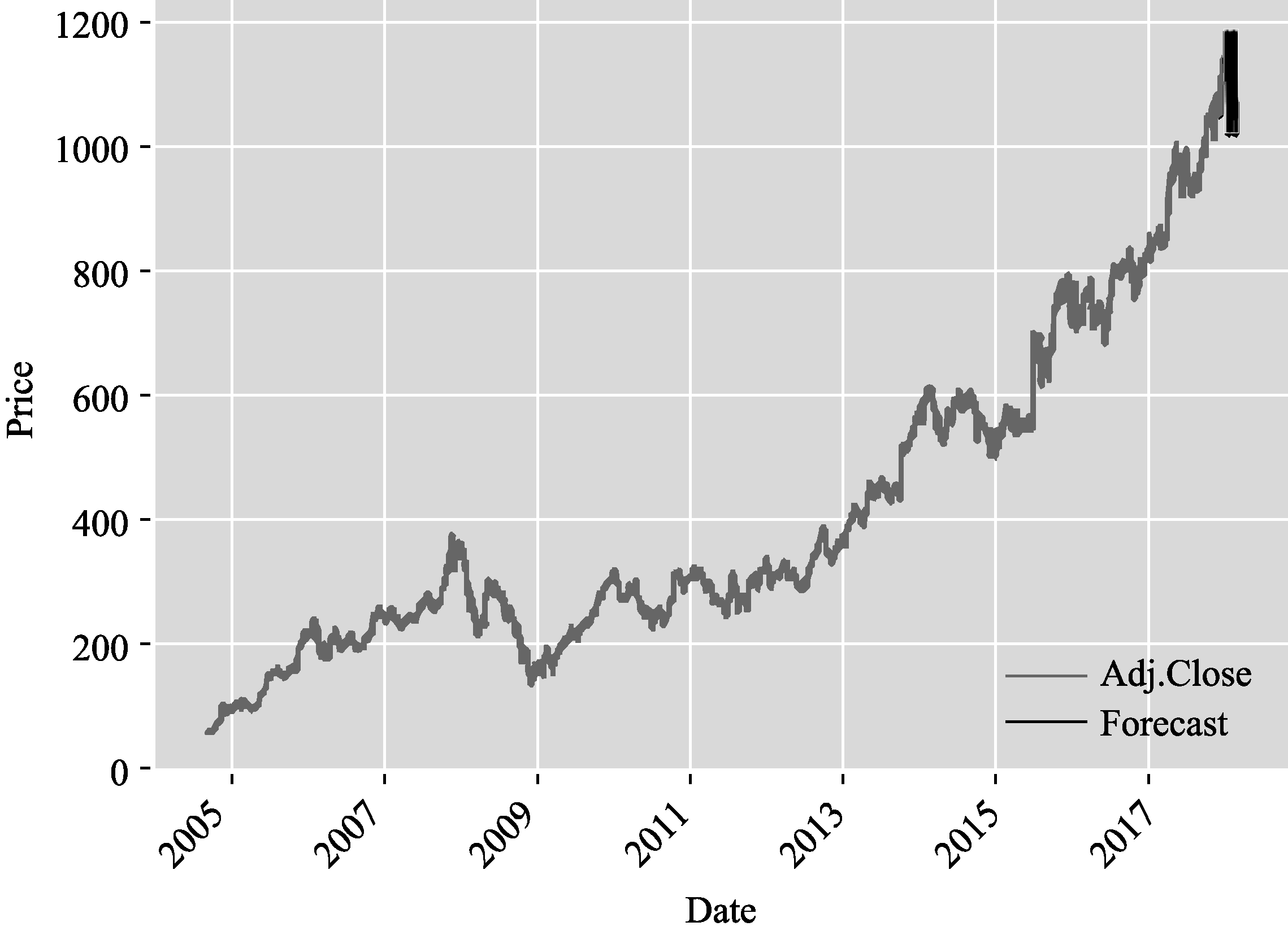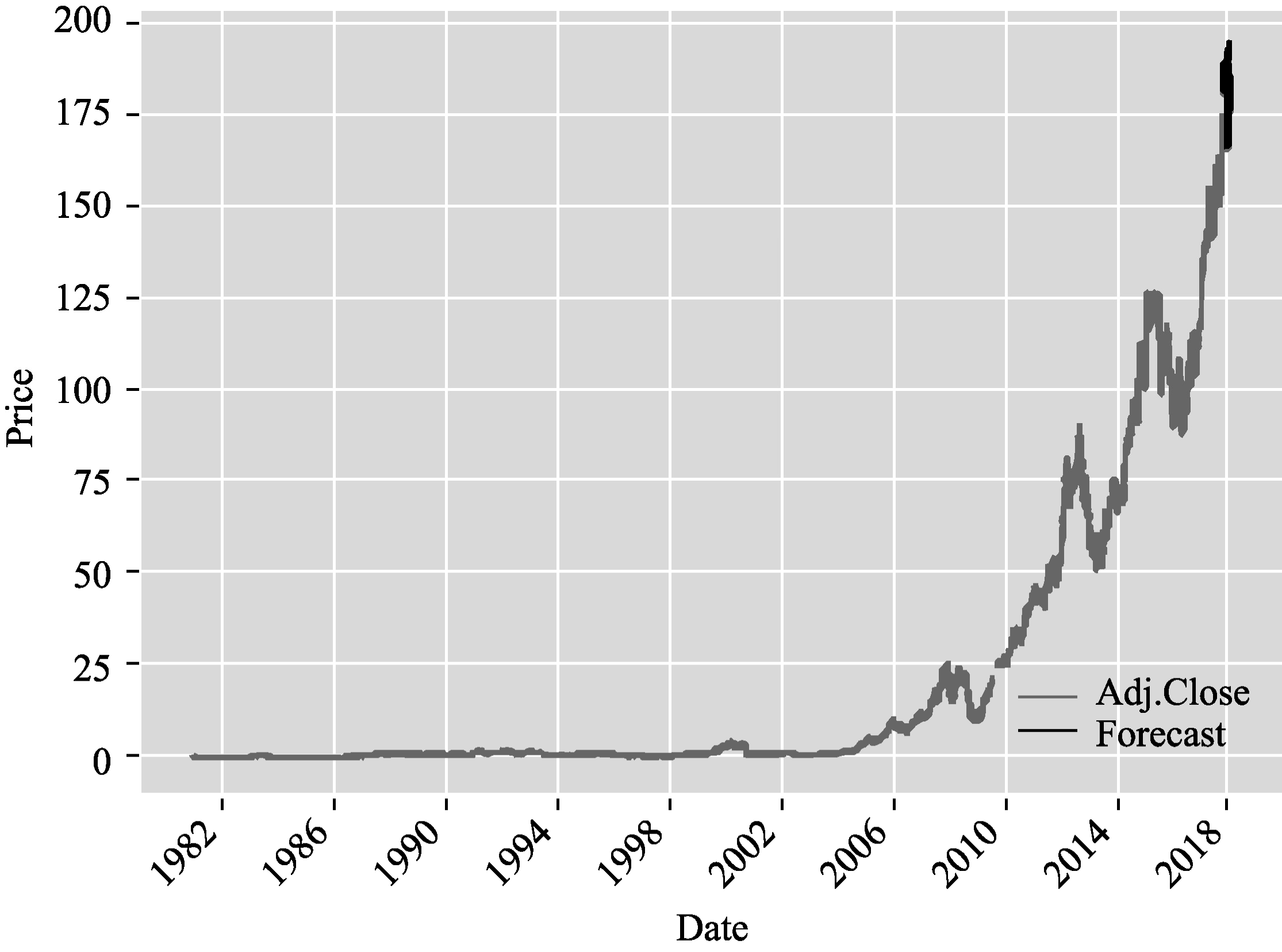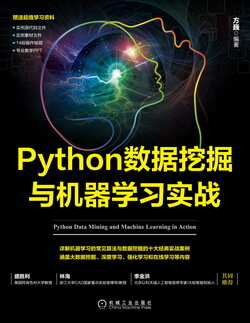2020-02-01 16:201632

## 评论

• ###### 【机器学习】拟合优度度量和梯度下降（红酒数据集的线性回归模型 sklearn&Ridge）

拟合度指回归直线与样本数据趋势的吻合程度。

2022-11-16

• ###### 39 丨数据挖掘实战（1）：信用卡违约率分析

当我们做数据挖掘的时候，如何选择最优分类算法呢？如何优化分类器的参数，得到更好的结果？

2019-03-13

• ###### 线性回归（下）：如何使用最小二乘法进行效果验证？

上一节我们讲了最小二乘法的核心思想和具体推导过程，今天我们就用实际的数据操练一下。

2019-03-20

• ###### 20 丨朴素贝叶斯分类（上）：如何让机器判断男女？

很多人都听说过贝叶斯原理，但记不住这些概率论的公式，没关系，我会尽量用通俗易懂的语言进行讲解。

2019-01-28

• ###### 携程如何基于 ARIMA 时序分析做业务量的预测

对于互联网公司而言，业务量是公司经营关注的重要指标之一。实际情况的复杂性给业务量的分析预测带来了许多挑战。

• ###### 模型的评估指标

如何对机器学习模型不同的性能进行度量呢？今天我们就来看看机器学习中模型的评估指标。

2018-06-21

• ###### 预测类产品（三）：从 0 打造一款“大白信用评分产品”

今天，我以小白信用评分产品为例，来教你模拟构建一个大白信用评分产品，从而学习到机器学习在互联网金融行业的产品落地方法。

2021-03-01

• ###### 基础线性回归：一元与多元

从今天开始，专栏将进入统计机器学习模块。虽然统计机器学习中千姿百态的模型让人眼花缭乱，但究其本原，它们都来源于最原始的线性回归。

2018-06-28

28天写作

Soul网关

Soul网关

io

#### 个人选择理财产品有哪些好方法